# Elegant regression results tables and plots in R: the finalfit package

May 16, 2018
By

Want to share your content on R-bloggers? click here if you have a blog, or here if you don't.

The `finafit` package brings together the day-to-day functions we use to generate final results tables and plots when modelling. I spent many years repeatedly manually copying results from R analyses and built these functions to automate our standard healthcare data workflow. It is particularly useful when undertaking a large study involving multiple different regression analyses. When combined with RMarkdown, the reporting becomes entirely automated. Its design follows Hadley Wickham’s tidy tool manifesto.

### Installation and Documentation

It lives on GitHub.

You can install `finalfit` from github with:

```# install.packages("devtools")
devtools::install_github("ewenharrison/finalfit")```

It is recommended that this package is used together with `dplyr`, which is a dependent.

Some of the functions require `rstan` and `boot`. These have been left as `Suggests` rather than `Depends` to avoid unnecessary installation. If needed, they can be installed in the normal way:

```install.packages("rstan")
install.packages("boot")```

To install off-line (or in a Safe Haven), download the zip file and use `devtools::install_local()`.

### Main Features

#### 1. Summarise variables/factors by a categorical variable

`summary_factorlist()` is a wrapper used to aggregate any number of explanatory variables by a single variable of interest. This is often “Table 1” of a published study. When categorical, the variable of interest can have a maximum of five levels. It uses `Hmisc::summary.formula()`.

```library(finalfit)
library(dplyr)

# Load example dataset, modified version of survival::colon
data(colon_s)

# Table 1 - Patient demographics by variable of interest ----
explanatory = c("age", "age.factor", "sex.factor", "obstruct.factor")
dependent = "perfor.factor" # Bowel perforation
colon_s %>%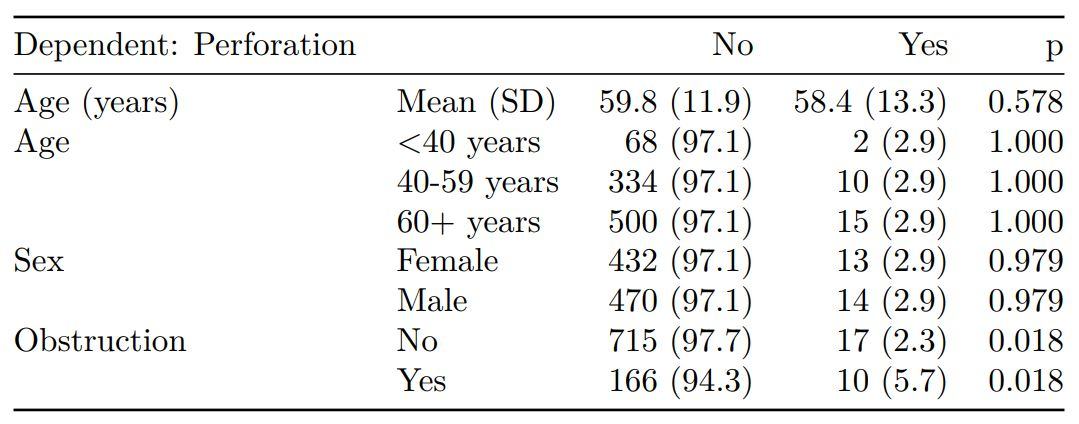See other options relating to inclusion of missing data, mean vs. median for continuous variables, column vs. row proportions, include a total column etc.

`summary_factorlist()` is also commonly used to summarise any number of variables by an outcome variable (say dead yes/no).

```# Table 2 - 5 yr mortality ----
explanatory = c("age", "age.factor", "sex.factor",
"obstruct.factor")
dependent = 'mort_5yr'
colon_s %>%Tables can be knitted to PDF, Word or html documents. We do this in RStudio from a .Rmd document. Example chunk:

``````{r, echo = FALSE, results='asis'}
knitr::kable(example_table, row.names=FALSE,
align=c("l", "l", "r", "r", "r", "r"))
``````

#### 2. Summarise regression model results in final table format

The second main feature is the ability to create final tables for linear (`lm()`), logistic (`glm()`), hierarchical logistic (`lme4::glmer()`) and
Cox proportional hazards (`survival::coxph()`) regression models.

The `finalfit()` “all-in-one” function takes a single dependent variable with a vector of explanatory variable names (continuous or categorical variables) to produce a final table for publication including summary statistics, univariable and multivariable regression analyses. The first columns are those produced by `summary_factorist()`. The appropriate regression model is chosen on the basis of the dependent variable type and other arguments passed.

##### Logistic regression: glm()

Of the form: `glm(depdendent ~ explanatory, family="binomial")`

```explanatory = c("age.factor", "sex.factor",
"obstruct.factor", "perfor.factor")
dependent = 'mort_5yr'
colon_s %>%
finalfit(dependent, explanatory)```##### Logistic regression with reduced model: glm()

Where a multivariable model contains a subset of the variables included specified in the full univariable set, this can be specified.

```explanatory = c("age.factor", "sex.factor", "obstruct.factor", "perfor.factor")
explanatory_multi = c("age.factor", "obstruct.factor")
dependent = 'mort_5yr'
colon_s %>%
finalfit(dependent, explanatory, explanatory_multi)```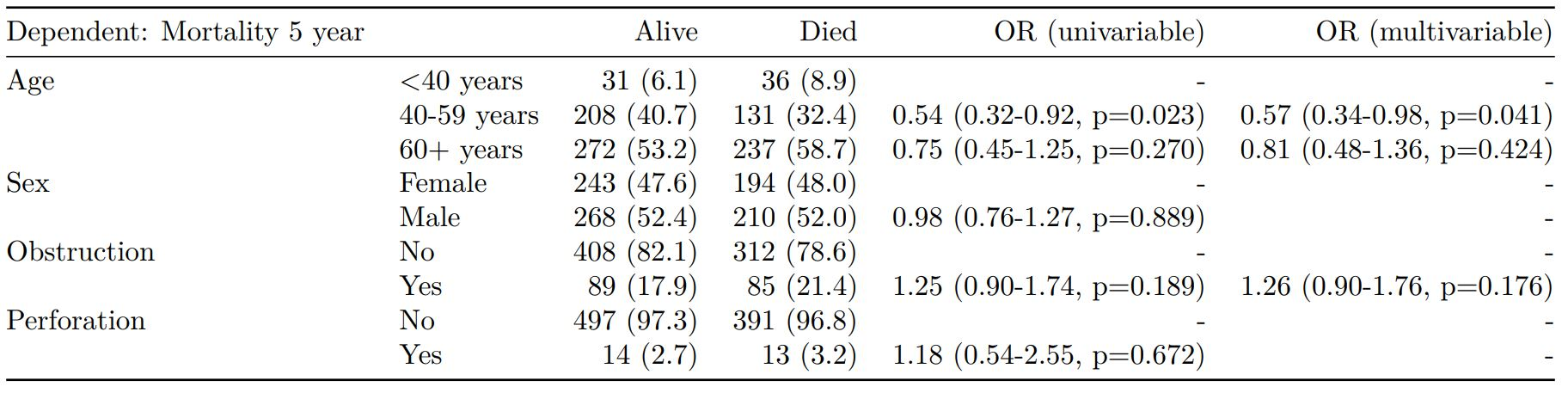##### Mixed effects logistic regression: lme4::glmer()

Of the form: `lme4::glmer(dependent ~ explanatory + (1 | random_effect), family="binomial")`

Hierarchical/mixed effects/multilevel logistic regression models can be specified using the argument `random_effect`. At the moment it is just set up for random intercepts (i.e. `(1 | random_effect)`, but in the future I’ll adjust this to accommodate random gradients if needed (i.e. `(variable1 | variable2)`.

```explanatory = c("age.factor", "sex.factor", "obstruct.factor", "perfor.factor")
explanatory_multi = c("age.factor", "obstruct.factor")
random_effect = "hospital"
dependent = 'mort_5yr'
colon_s %>%
finalfit(dependent, explanatory, explanatory_multi, random_effect)```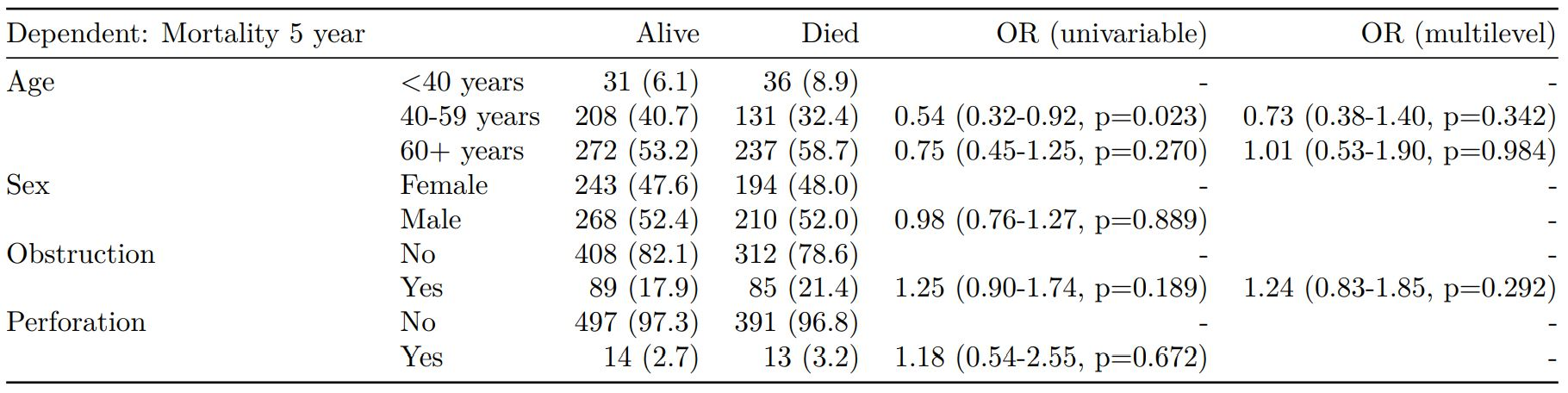##### Cox proportional hazards: survival::coxph()

Of the form: `survival::coxph(dependent ~ explanatory)`

```explanatory = c("age.factor", "sex.factor", "obstruct.factor", "perfor.factor")
dependent = "Surv(time, status)"
colon_s %>%
finalfit(dependent, explanatory)```##### Add common model metrics to output

`metrics=TRUE` provides common model metrics. The output is a list of two dataframes. Note chunk specification for output below.

```explanatory = c("age.factor", "sex.factor", "obstruct.factor", "perfor.factor")
dependent = 'mort_5yr'
colon_s %>%
finalfit(dependent, explanatory, metrics=TRUE)```

``````{r, echo=FALSE, results="asis"}
knitr::kable(table7[], row.names=FALSE, align=c("l", "l", "r", "r", "r"))
knitr::kable(table7[], row.names=FALSE)
``````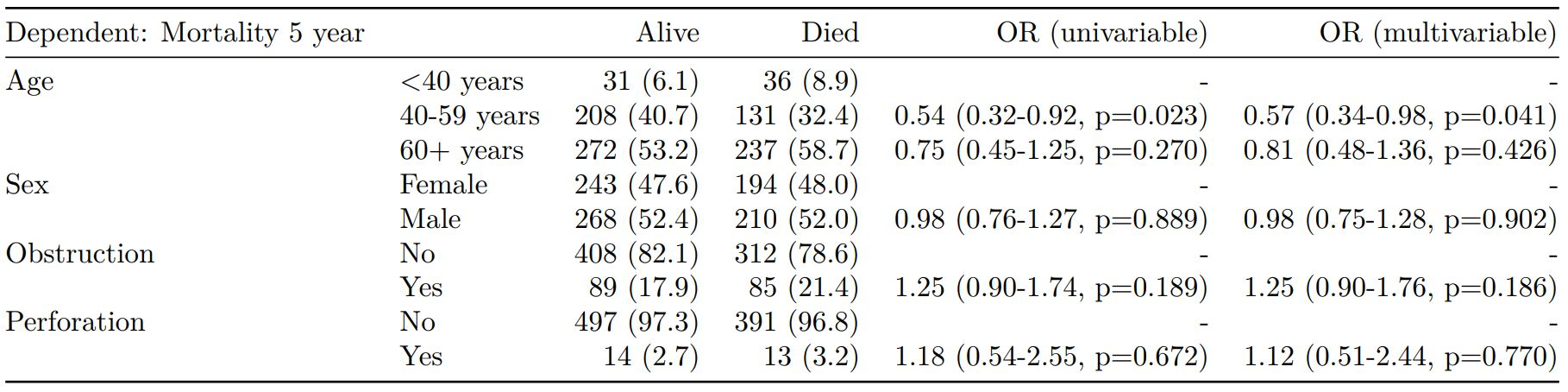Rather than going all-in-one, any number of subset models can be manually added on to a `summary_factorlist()` table using `finalfit_merge()`. This is particularly useful when models take a long-time to run or are complicated.

Note the requirement for `fit_id=TRUE` in `summary_factorlist()`. `fit2df` extracts, condenses, and add metrics to supported models.

```explanatory = c("age.factor", "sex.factor", "obstruct.factor", "perfor.factor")
explanatory_multi = c("age.factor", "obstruct.factor")
random_effect = "hospital"
dependent = 'mort_5yr'

# Separate tables
colon_s %>%
summary_factorlist(dependent, explanatory, fit_id=TRUE) -> example.summary

colon_s %>%
glmuni(dependent, explanatory) %>%
fit2df(estimate_suffix=" (univariable)") -> example.univariable

colon_s %>%
glmmulti(dependent, explanatory) %>%
fit2df(estimate_suffix=" (multivariable)") -> example.multivariable

colon_s %>%
glmmixed(dependent, explanatory, random_effect) %>%
fit2df(estimate_suffix=" (multilevel)") -> example.multilevel

# Pipe together
example.summary %>%
finalfit_merge(example.univariable) %>%
finalfit_merge(example.multivariable) %>%
finalfit_merge(example.multilevel) %>%
select(-c(fit_id, index)) %>% # remove unnecessary columns
dependent_label(colon_s, dependent, prefix="") # place dependent variable label```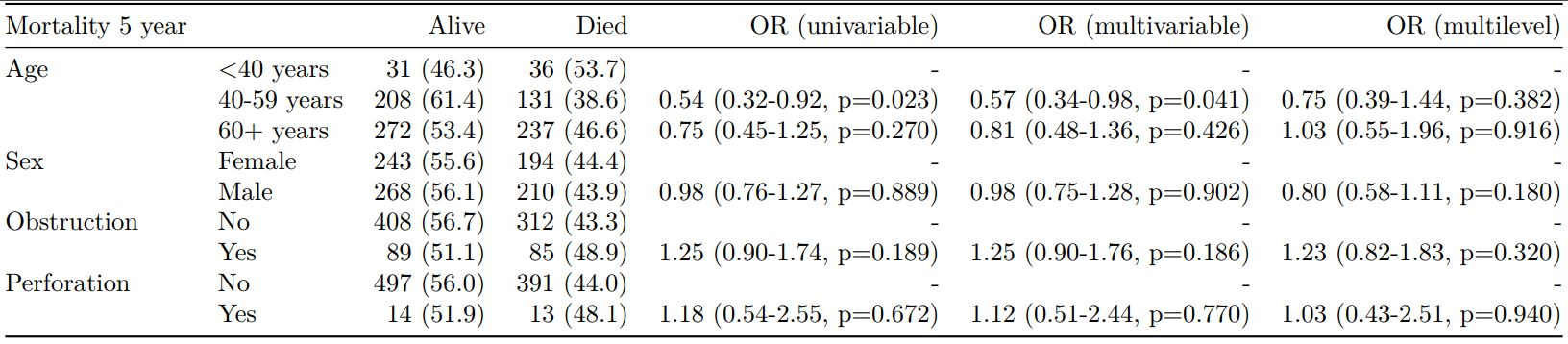##### Bayesian logistic regression: with `stan`

Our own particular `rstan` models are supported and will be documented in the future. Broadly, if you are running (hierarchical) logistic regression models in [Stan](http://mc-stan.org/users/interfaces/rstan) with coefficients specified as a vector labelled `beta`, then `fit2df()` will work directly on the `stanfit` object in a similar manner to if it was a `glm` or `glmerMod` object.

### 3. Summarise regression model results in plot

Models can be summarized with odds ratio/hazard ratio plots using `or_plot`, `hr_plot` and `surv_plot`.

#### OR plot

```# OR plot
explanatory = c("age.factor", "sex.factor", "obstruct.factor", "perfor.factor")
dependent = 'mort_5yr'
colon_s %>%
or_plot(dependent, explanatory)
# Previously fitted models (`glmmulti()` or `glmmixed()`) can be provided directly to `glmfit````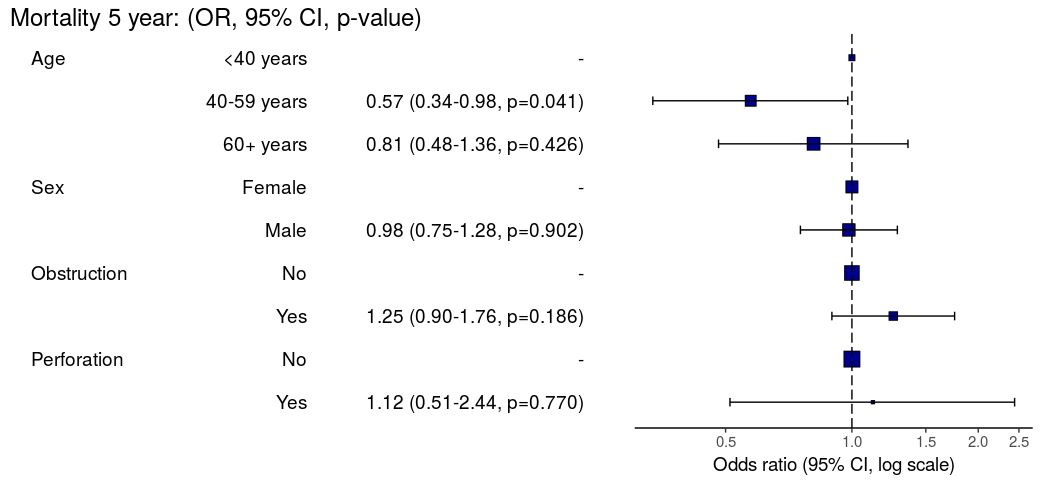#### HR plot

```# HR plot
explanatory = c("age.factor", "sex.factor", "obstruct.factor", "perfor.factor")
dependent = "Surv(time, status)"
colon_s %>%
hr_plot(dependent, explanatory, dependent_label = "Survival")
# Previously fitted models (`coxphmulti`) can be provided directly using `coxfit````#### Kaplan-Meier survival plots

KM plots can be produced using the `library(survminer)`

```# KM plot
explanatory = c("perfor.factor")
dependent = "Surv(time, status)"
colon_s %>%
surv_plot(dependent, explanatory, xlab="Time (days)", pval=TRUE, legend="none")```### Notes

Use `Hmisc::label()` to assign labels to variables for tables and plots.

`label(colon_s\$age.factor) = "Age (years)"`

Export dataframe tables directly or to R Markdown `knitr::kable()`.

Note wrapper `summary_missing()` is also useful. Wraps `mice::md.pattern`.

```colon_s %>%
summary_missing(dependent, explanatory)```

Development will be on-going, but any input appreciated.

R-bloggers.com offers daily e-mail updates about R news and tutorials about learning R and many other topics. Click here if you're looking to post or find an R/data-science job.
Want to share your content on R-bloggers? click here if you have a blog, or here if you don't.